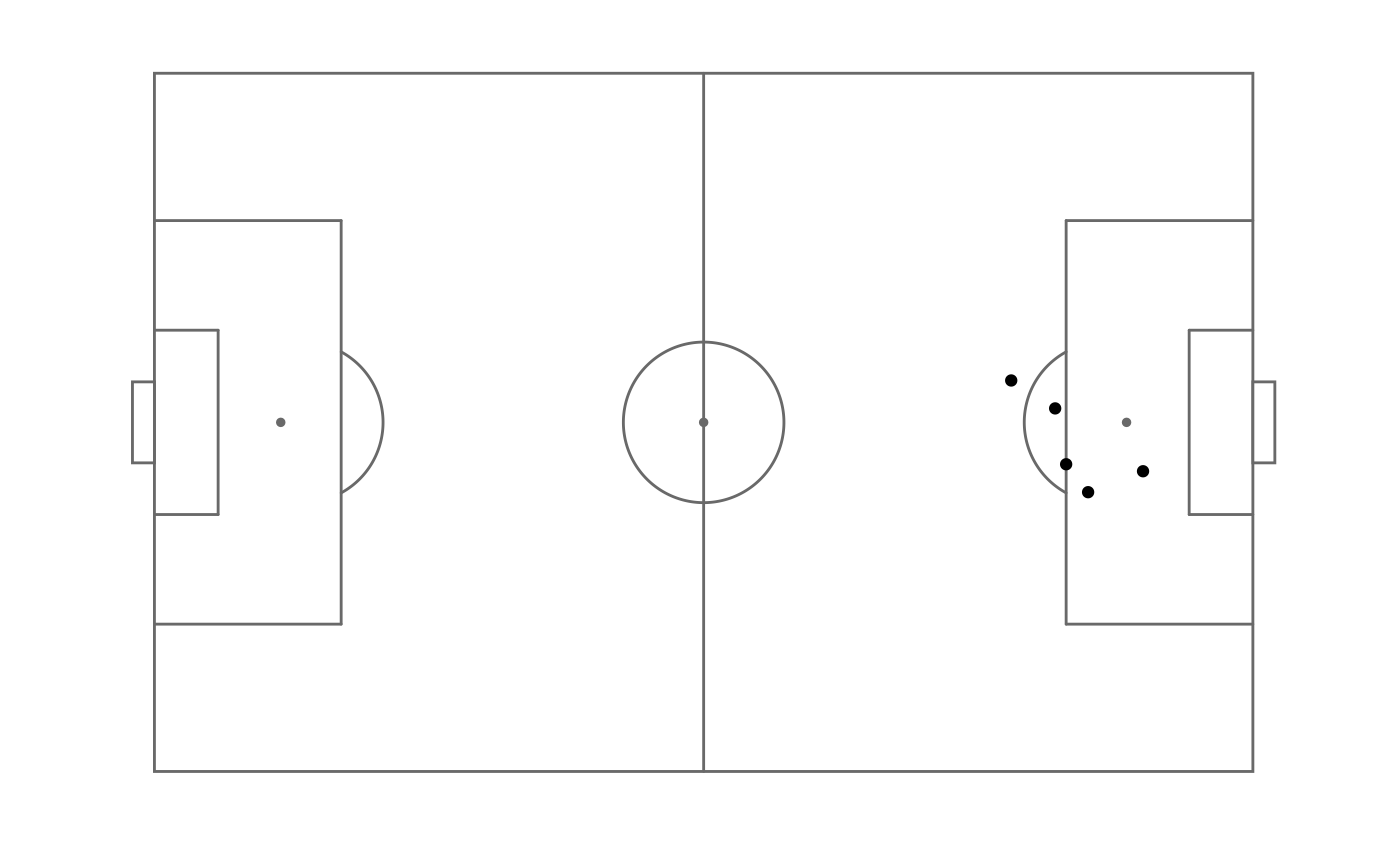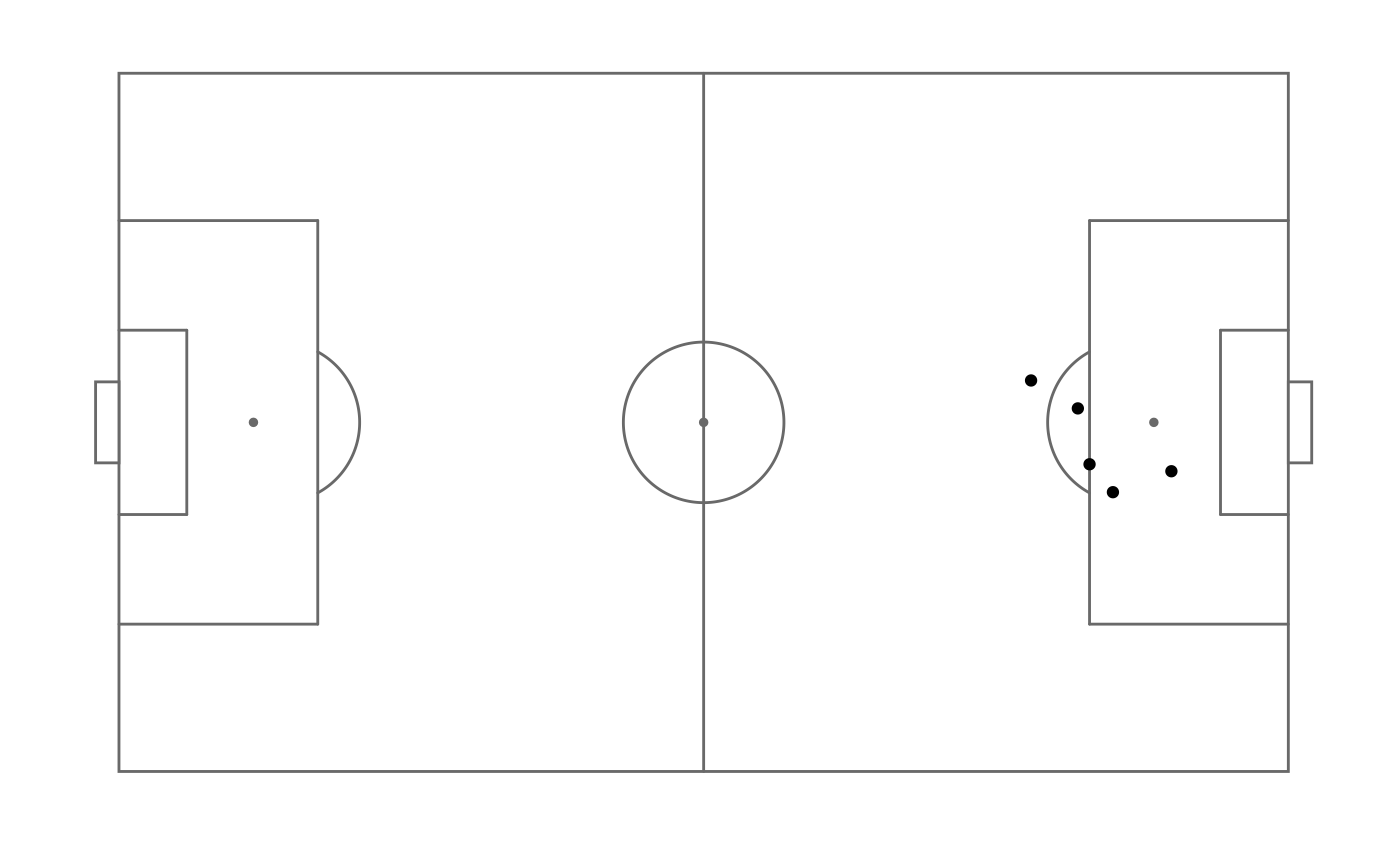Functionally very similar to ggplot2::theme_void.

theme_pitch(aspect_ratio = 68/105)

Arguments

aspect_ratio

Aspect ratio (y / x) for the plot. Use NULL to let the plot take any aspect ratio.

Value

list of ggplot themes to be added to a ggplot plot

Examples

shots_data <- data.frame(x = c(90, 85, 82, 78, 83),
y = c(43, 40, 52, 56, 44))

p <- ggplot(shots_data, aes(x = x, y = y)) +

# Pitch fixed to 68/105 by default
p + theme_pitch()# Free aspect
p + theme_pitch(aspect_ratio = NULL)